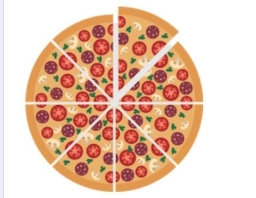# Time vs pizza

If Mr. Cromleigh can eat 1/4 of a pizza in 1/6 of an hour, how many pizzas can he eat in one hour?

He can eat ____ pizzas.

x =  3/2 = 1 12

### Step-by-step explanation:Did you find an error or inaccuracy? Feel free to write us. Thank you!

Tips for related online calculators
Need help calculating sum, simplifying, or multiplying fractions? Try our fraction calculator.
Do you want to convert time units like minutes to seconds?